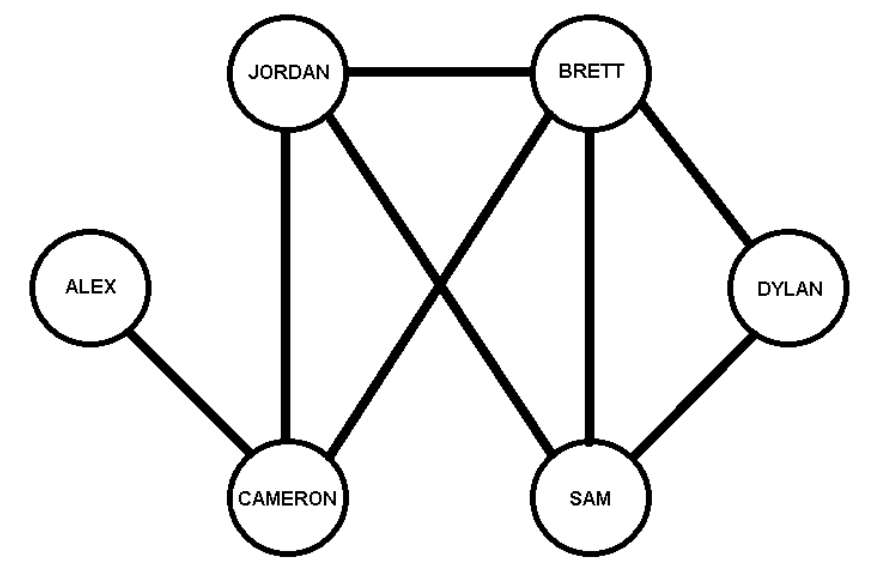시간 제한메모리 제한제출정답맞힌 사람정답 비율
2 초 512 MB111100.000%

## 문제

In many networks, we need a measure of the closeness of one node to another. The classic measure is link distance. If the network is represented as a connected undirected graph with an edge between any pair of nodes that have a direct link, the link distance is the number of edges in the shortest path between the nodes. For example, the Kevin Bacon number of an actor is the link distance from the actor to Kevin Bacon in a graph with nodes actors and an edge between two actors if they have appeared in the same movie. The Erdos number for mathematicians is the link distance from a mathematician to Paul Erdos in a graph with nodes mathematicians and an edge between two mathematicians if they have co-authored a published paper. In the graph below, the link distance from ALEX to JORDAN is 2 and the link distance from ALEX to SAM or DYLAN is 3.In the graph, the link distance from JORDAN to ALEX or to DYLAN is the same (2) but JORDAN and DYLAN are in some sense “more connected” than JORDAN and ALEX. An attempt to reflect this in a distance measurement is resistance distance. This is similar to the relationship between friends in online social networks. In this case, the nodes in the graph are people (as shown above) and the resistance distance between them is the closeness of their friendship.

The resistance distance between two nodes in a graph is computed by finding the resistance between the two nodes in the graph as an electrical network with 1 ohm resistors for each edge. That is: if node 1 is held at V1 and node 2 is held at V2, (V2 != V1), and the voltages at all other nodes are allowed to float, the resistance is (V1 – V2) / (current from node 1 to node 2). Or, the resistance is (V1 – V2) when the current from node 1 to node 2 is one ampere.

Recall:

1. The sum of the currents on edges into a node must be the current flowing out to the external world (-1 for node 1, 1 for node 2 and 0 for all other nodes).
2. The voltage drop across an edge from node a to node b is the current in the edge times the resistance along the edge (which is 1 in our case).

For this problem, you will write a program which takes as input the description of a graph as nodes and edges and a set of pairs of nodes in the graph and computes the resistance distance between each pair of nodes in the list. The sample Input/Output below computes the resistance distance from node JORDAN to each other node in the picture above.

## 입력

The first line of input contains a single decimal integer P, (1 ≤ P ≤ 10000), which is the number of data sets that follow. Each data set should be processed identically and independently.

Each data set consists of multiple lines of input. The first line of each data set contains the data set number, K, the number of nodes, N (2 ≤ N ≤ 20), the number of queries, Q (1 ≤ Q ≤ 10) and the number of edges, E (1 ≤ E ≤ N(N-1)/2). This line is followed by a set of lines describing the edges and then a set of lines giving a list of resistance distances to compute for the graph.

The edges are described by a sequence of lines containing a node number, n (1 ≤ n ≤ N), a count and the nodes which are connected to node n. The sequence ends when E edges have been specified. There will be no self-loops or parallel edges.

The queries are described by Q lines, each of which has a query number, q (1 ≤ q ≤ Q), and two node numbers n1 and n2. The program is to find the resistance distance from n1 to n2.

## 출력

For each data set there is one line of output. The single output line consists of the data set number, K, followed by a single space followed by the queried resistance distances to 3 decimal places in the order of the input.

## 예제 입력 1

1
1 6 5 8
3 3 1 2 4
4 3 2 5 6
5 2 2 6
1 2 1
2 2 3
3 2 4
4 2 5
5 2 6


## 예제 출력 1

1 1.619 0.619 0.476 0.571 0.905